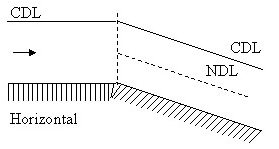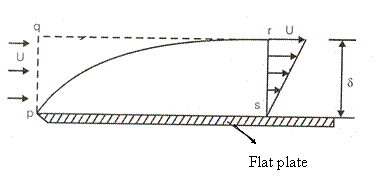# Fluid Mechanics - 2

## 20 Questions MCQ Test GATE Mechanical (ME) 2023 Mock Test Series | Fluid Mechanics - 2

Description
Attempt Fluid Mechanics - 2 | 20 questions in 60 minutes | Mock test for GATE preparation | Free important questions MCQ to study GATE Mechanical (ME) 2023 Mock Test Series for GATE Exam | Download free PDF with solutions
QUESTION: 1

### Two pipe systems are said to be equivalent when

Solution:
*Answer can only contain numeric values
QUESTION: 2

Solution:
QUESTION: 3

### Water (S = 1, μ = 1 centipoise) flows under pressure through of a pipe of 1 cm diameter at a velocity of 60 cm/min. What would be the head loss per km length of the pipe?

Solution:
QUESTION: 4

The Dray-Weisbach equation for head loss is valid

Solution:
QUESTION: 5

For a solid sphere falling under gravity at terminal velocity in a fluid.

Solution:
QUESTION: 6

For uniform flow in a channel

Solution:
QUESTION: 7

The relationship between Manning’s coefficient ‘n’ and Chezy’s coefficient 'c' is given by

Solution:
QUESTION: 8

If the specific energy at the upstream section of a rectangular channel is 3 m and minimum specific energy is 2.5 m, the maximum height of hump without causing afflux will be

Solution:
QUESTION: 9

For a steep slope profile S1, the type of flow will be

Solution:
QUESTION: 10

Pick up the incorrect statement with respect to critical flow

Solution:
QUESTION: 11

The critical depth of flow in a most economical triangular channel section for a discharge of 1 m 3/sec is given by

Solution:
QUESTION: 12

If the depth of flow in a channel is 1 m and velocity of flow is 2 m/sec. then the velocity with which an elementary wave can travel down stream

Solution:
QUESTION: 13

Figure below shoes gradually varied flow in an open chennal with a brake in bed slopeTypes of water surface profiles occuring from left to right is

Solution:
QUESTION: 14

Which one of the following pairs of situations and types of water surface profiles is not correctly matched?

Solution:
QUESTION: 15

A discharge of 3.0 m3/s flows in a canal, 2 m side, at a depth of 1.2 m. If the width of the canal is reduced to 1.5 m by a canal transition, then the neglecting losses, the depth of flow after the contraction will be

Solution:
QUESTION: 16

A hydraulically efficient trapezoidal section of open channel flow carries water at the optimal depth of 0.6m.Chezy coefficient is 75 and bed slope is 1 in 250. What is the discharge through the channel?

Solution:
QUESTION: 17

Common Data Questions (32 and 33):
A smooth flat plate with a sharp leading edge is placed along a gas free stream flowing at U = 10 m/s. The thickness of the boundary layer at section r-s is 10 mm, the breadth of the plate is 1 m (into the paper) and the density of the gas, p = 1.0 kg/m3 . Assume that the boundary layer is thin, two - dimensional, and follows a linear velocity distribution, u = U (y/δ), at the section r-s, where y is the height from plate.Q. The mass flow rate in Kg/s accros the section q-r is

Solution:
QUESTION: 18

A smooth flat plate with a sharp leading edge is placed along a gas free stream flowing at U = 10 m/s. The thickness of the boundary layer at section r-s is 10 mm, the breadth of the plate is 1 m (into the paper) and the density of the gas, p = 1.0 kg/m3 . Assume that the boundary layer is thin, two - dimensional, and follows a linear velocity distribution, u = U (y/δ), at the section r-s, where y is the height from plate.Q. The integrated drag force in N on the plate, between p-s, is

Solution:
QUESTION: 19

Linked Data Questions (34 and 35):
Consider fully developed, laminar flow in a circular pipe of radius ‘R’ .The centre - line velocity of the flow is Um.

Q. The velocity u at a radial distance r from the center line of the pipe is given by

Solution:
QUESTION: 20

Consider fully developed, laminar flow in a circular pipe of radius ‘R’ .The centre - line velocity of the flow is Um

Q.The value of the radial distance from the centre line of the pipe at which the velocity would be equal to the average velocity of the flow is

Solution:Use Code STAYHOME200 and get INR 200 additional OFF Use Coupon Code